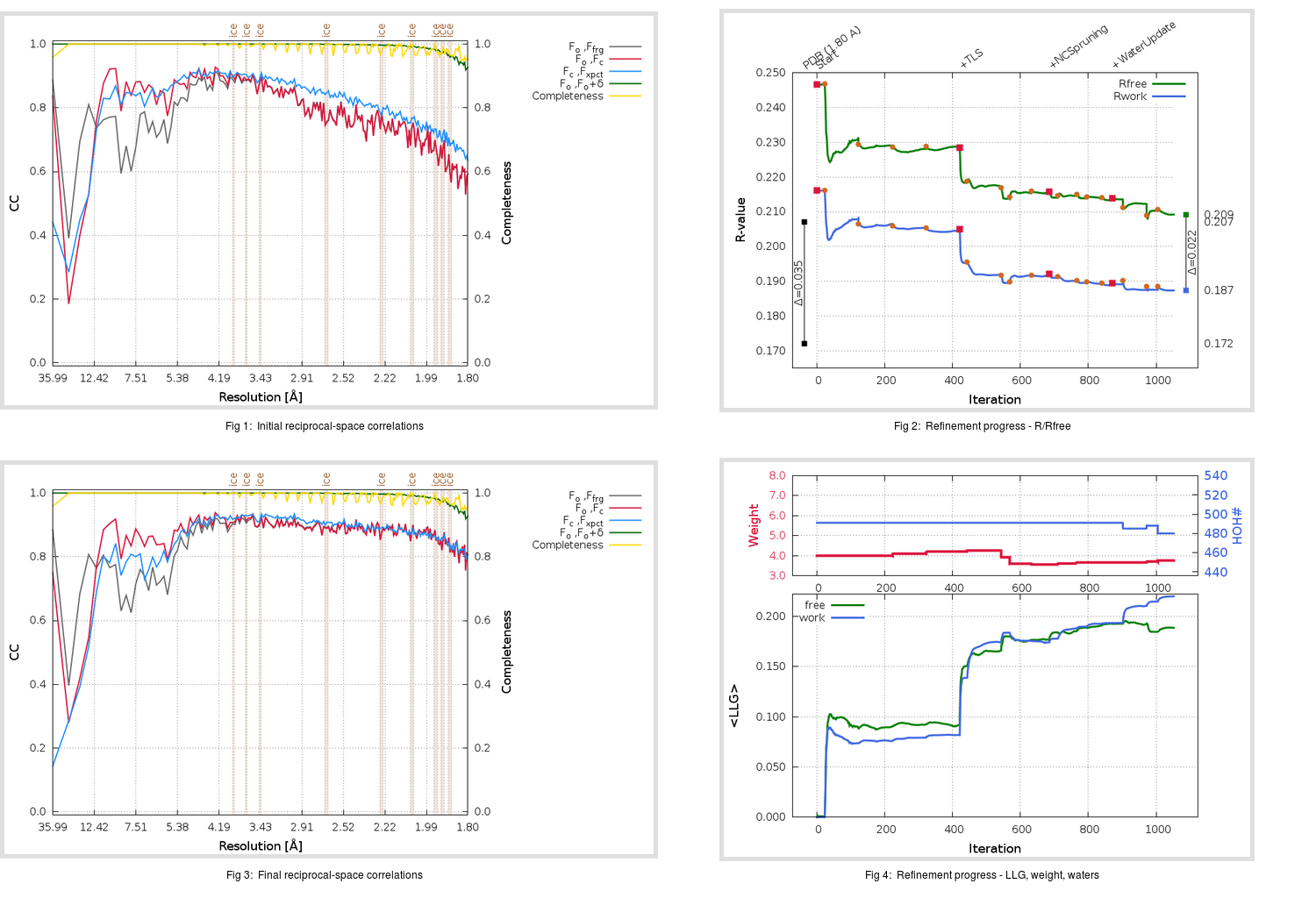Content:

```    Diffraction limits & principal axes of ellipsoid fitted to diffraction cut-off surface:
1.766         0.9382   0.0000  -0.3460       0.795 a* - 0.606 c*
1.774         0.0000   1.0000   0.0000       b*
1.773         0.3460   0.0000   0.9382       0.304 a* + 0.953 c*
```

## Deposited

` `
 Date deposited Date data collection Resolution R, Rfree 20070127 20040613 1.80 0.1700 0.2070

Molprobity (CCP4 7.0 version) summary:

```Ramachandran outliers =   0.17 %
favored =  97.65 %
Rotamer outliers      =   4.66 %
C-beta deviations     =     4
Clashscore            =   8.06
RMS(bonds)            =   0.0287
RMS(angles)           =   1.65
MolProbity score      =   2.02
Resolution            =   1.80
R-work                =   0.1700
R-free                =   0.2070
```

```Number of waters      =   491

<B> (all atoms) =   47.22 ( sd =    7.82 ) for       5198 non-hydrogen atoms
<B>   (protein) =   46.64 ( sd =    7.03 ) for       4649 non-hydrogen atoms
<B>     (water) =   52.28 ( sd =   12.12 ) for        491 non-hydrogen atoms
<B>    (others) =   50.60 ( sd =    3.77 ) for         58 non-hydrogen atoms

B min/max       (all non-hydrogen atoms) =   25.68 /  100.08
B min/max   (protein non-hydrogen atoms) =   33.08 /   78.71
B min/max     (water non-hydrogen atoms) =   25.68 /  100.08
B min/max     (other non-hydrogen atoms) =   40.50 /   57.75
```

## BUSTER (re-)refinement

` `

Molprobity (CCP4 7.0 version) summary:

```Ramachandran outliers =   0.17 %
favored =  97.64 %
Rotamer outliers      =   3.88 %
C-beta deviations     =     0
Clashscore            =   2.90
RMS(bonds)            =   0.0118
RMS(angles)           =   1.57
MolProbity score      =   1.60
Resolution            =   1.80
R-work                =   0.1874
R-free                =   0.2093
```

```Number of waters      =   480

<B> (all atoms) =   34.55 ( sd =   12.05 ) for       5187 non-hydrogen atoms
<B>   (protein) =   33.00 ( sd =   11.08 ) for       4649 non-hydrogen atoms
<B>     (water) =   47.10 ( sd =   11.92 ) for        480 non-hydrogen atoms
<B>    (others) =   55.45 ( sd =    5.58 ) for         58 non-hydrogen atoms

B min/max       (all non-hydrogen atoms) =   15.06 /   98.38
B min/max   (protein non-hydrogen atoms) =   15.06 /   78.99
B min/max     (water non-hydrogen atoms) =   20.32 /   98.38
B min/max     (other non-hydrogen atoms) =   43.39 /   63.37
```

Refinement progression:Results:

` `
 File Remark 2OP9_aB_refine.01_04_refine.pdb.gz exact refinement commands are in header 2OP9_aB_refine.01_04_refine.mtz.gz including original deposited data and several re-refinement map coefficients 2OP9_aB_refine.01_04_BUSTER_model.cif.gz including any non-standard compound restraints 2OP9_aB_refine.01_04_BUSTER_refln.cif.gz